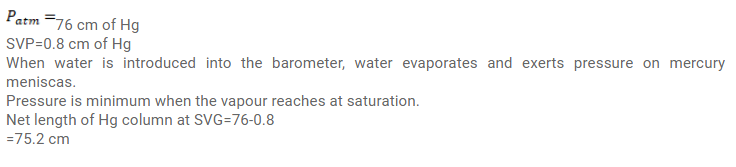# Use R=8.3 J/mol-K wherever required. A barometer correctly reads the atmosphericQuestion:

Use $\mathrm{R}=8.3 \mathrm{~J} / \mathrm{mol}-\mathrm{K}$ wherever required.

A barometer correctly reads the atmospheric pressure as $76 \mathrm{~cm}$ of mercury. Water droplets are slowly introduced into the barometer tube by a dropper. The height of the mercury column first decreases and then becomes constant. If the saturation vapour pressure at atmospheric temperature is $0.80 \mathrm{~cm}$ of mercury, find the height of the mercury column when it reaches its minimum value.

Solution: